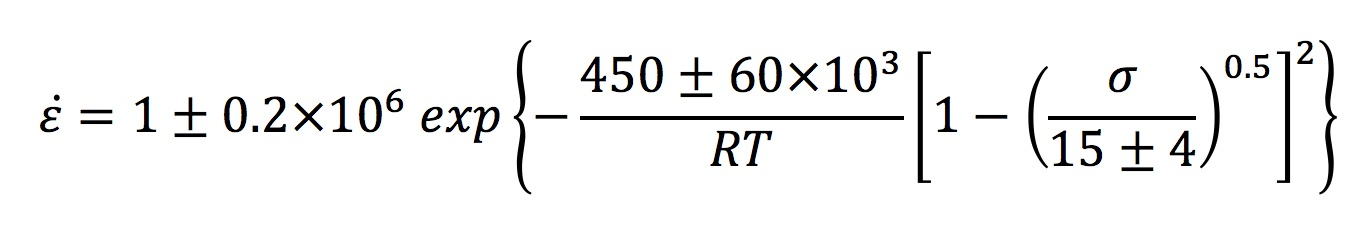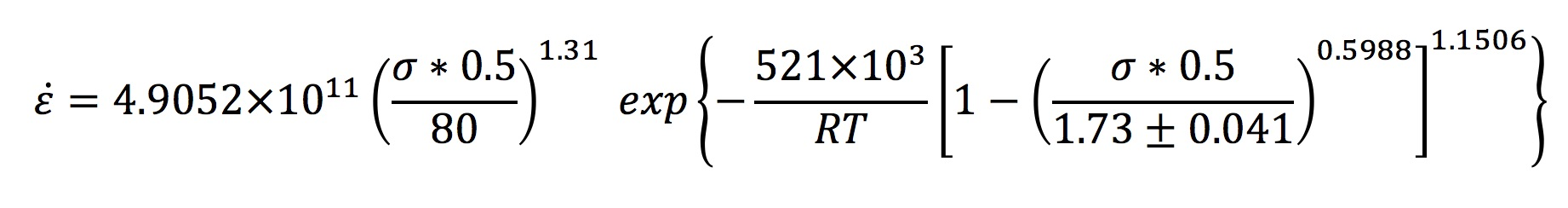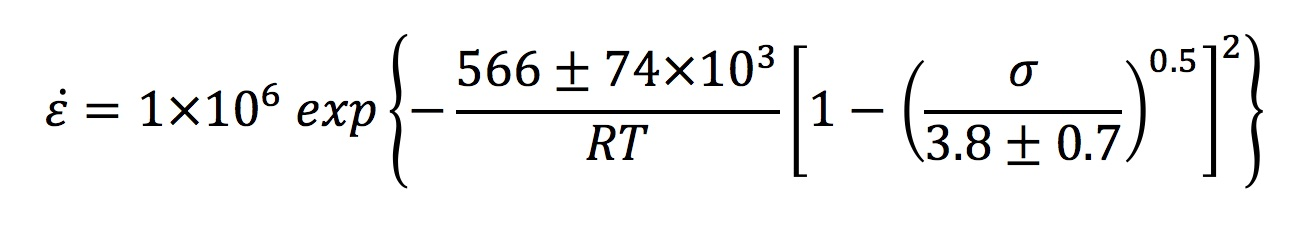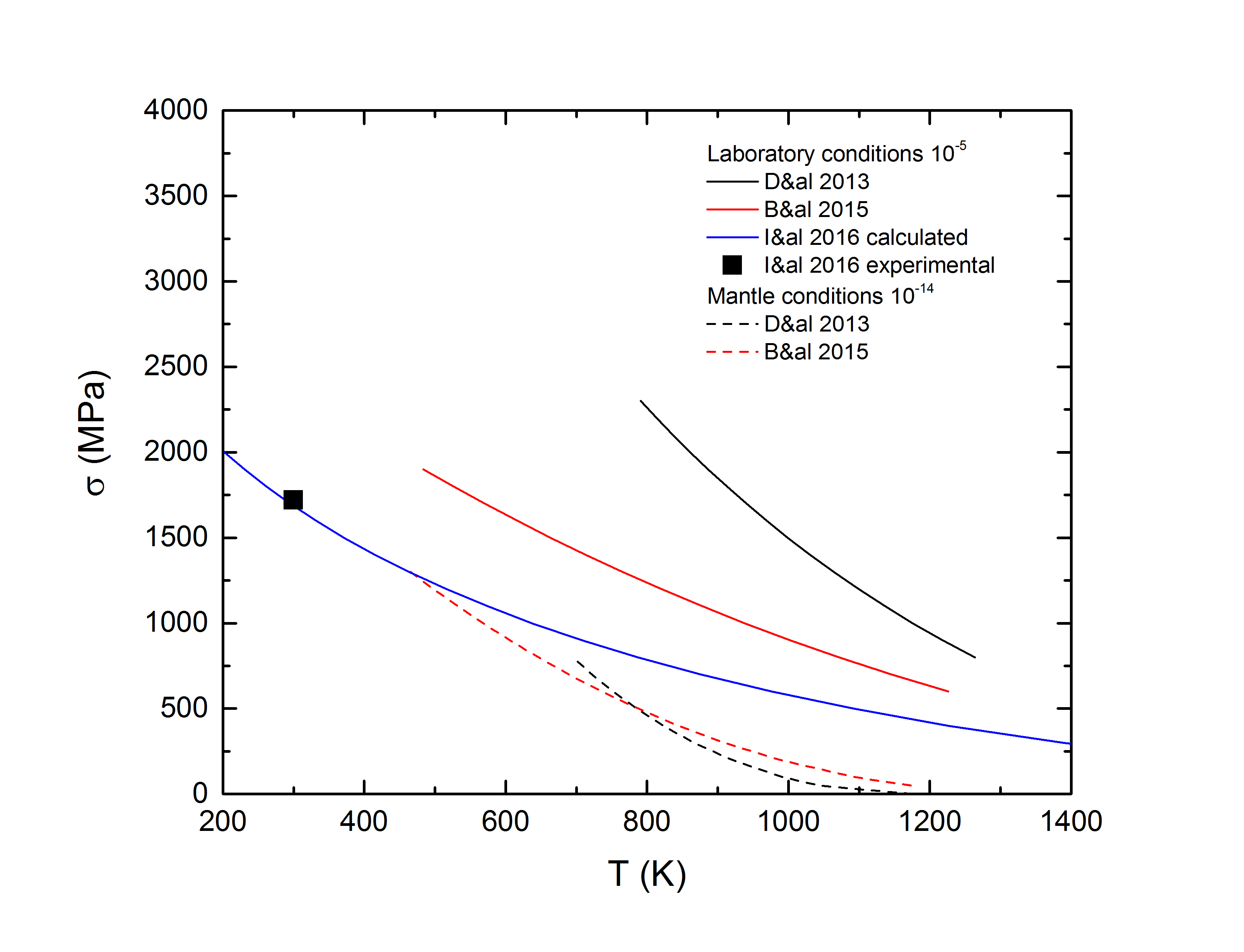# Olivine flow laws

Based on the experimental and numerical results on olivine deformation between 500 K and 1400 K, several semi-empirical exponential flow laws have been established for the uppermost upper mantle:where T is the absolute temperature, R is the gas constant, and σ is the applied (differential) stress in GPa

Domain of validity: temperature range [800K ; 1300K] and applied stress in the range [88 ; 754 MPa].where T is the absolute temperature, R is the gas constant, and σ is the applied stress reduced by the Schmid factor in GPa

Domain of validity: temperature range [800K ; 1973K] and applied stress in the range [50 ; 500 MPa].where T is the absolute temperature, R is the gas constant, and σ is the flow stress in GPa.

Domain of validity: temperature range [300K ; 1200K] and applied stress in the range [10 ; 2000 MPa].